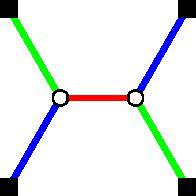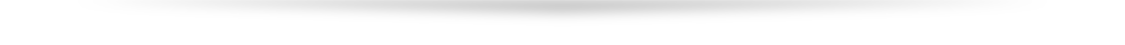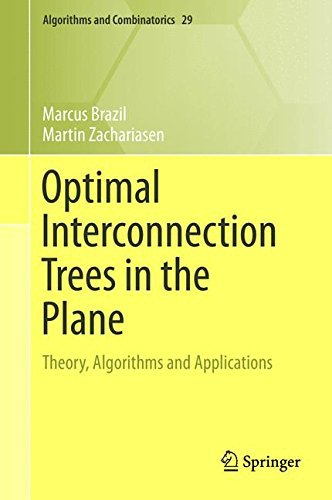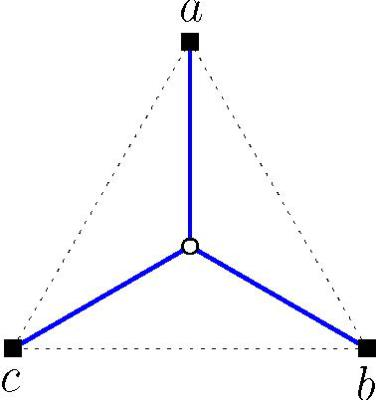The Steiner Tree PageSteiner Trees

### The mathematics of optimal interconnection networksThis website is an accompaniment to the book "Optimal Interconnection Trees in the Plane"  by Marcus Brazil and Martin Zachariasen and published by Springer.

The book explores fundamental aspects of geometric network optimisation, with applications to a variety of real world problems. It is aimed a graduate students, mathematicians, engineers and computer scientists. The book explains the principles required for designing interconnection networks in the plane that are as cost efficient as possible.

This website includes information about the authors, supplementary teaching  materials, and notes on the book.

The book can be purchased directly from the publishers at
What is a Steiner tree?
Suppose you are asked to design a system of roads to connect a number of towns. For simplicity, we assume that the location of each town can be represented by a single point, and that the area around the towns is completely flat with no obstacles. To keep costs low, you want the total length of all the roads to be as small as possible. This shortest length road network is an example of a minimum Steiner tree.
If there are only 2 towns, then the best solution is to build a single straight-line road between them. However, if there are 3 towns then the best solution generally involves placing a junction between the 3 towns and building straight roads from the junction to each town.Here is an example of a minimum Steiner tree for 3 towns labelled a, b and c. The problem of finding such a network is called the Steiner tree problem. As the number of towns to be connected increases this problem becomes increasingly difficult to solve.
Why study the Steiner tree problem?
• Minimum Steiner trees exhibit an elegant geometric structure that allows the Steiner tree problem to be solved through a combination of geometry and combinatorics. Even though the problem is intrinsically hard (it is an example of an NP-hard problem) the mathematical theory can be used to design surprisingly efficient algorithms for exactly solving the problem.

• This attractive combination of geometric structure and practical exact algorithms makes the Steiner tree problem a perfect introduction to the field of Computational Geometry.

• Steiner trees also have numerous applications in areas ranging from the physical design of microchips to relay placement in wireless sensor networks to tunnel layout in the design of underground mines.